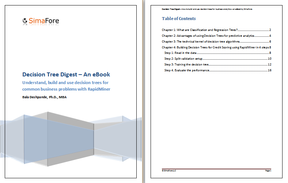Contact us - 262-780-0962

### Subscribe to Email Updates

A frequent situation encountered in classification problems is that of unbalanced data. A training dataset consisting of a disprortionately high number of examples from one class will result in a classifier that is biased towards this majority class. When a classification algorithm trained on such data is applied to a test dataset that is also unbalanced, the classifier will yield a very optimistic accuracy estimate. This phenomenon is very common in binary classification. The plot below shows an example of imbalanced data: the "positive" class indicated by blue is disproportionately higher than the "negative" class in red.What is the effect of data imbalance?

Let us explore this using a simple example. The data set shown in the process below is available in RapidMiner's Samples repository and called "Weighting". This is a balanced data set consisting of about 500 examples with the label variable consisting of roughly 50% "positive" and 50% "negative" classes respectively. Thus it is a balanced data set. When we train a decision tree to classify this data, we get an overall accuracy of 84%. The main thing to note here is that the decision tree accuracy on both the classes are roughly the same ~ 80%. See graphic below.As stated earlier, if we use unbalanced data to train a classifier, prediction will be biased towards the more frequent class. We now introduce a sub process called "Unbalance" which will resample the original data to introduce a skew: the resulting data set has more "positive" class examples than "negative" class examples. Specifically, we now have a data set with 92% belonging to the positive class and 8% belonging to negative class. The process and the results are shown below.How to address data imbalance?

There are several ways to fix this situation. The most commonly used method is to resample the data to restore the balance. This involves undersampling the more frequent class - in our case, the "positive" class and oversampling the less frequent "negative" class.

The "rebalance" sub process achieves this in our final RapidMiner process. As seen below, the overall accuracy is now back to the level of the original balanced data. The decision tree also looks a little bit similar to the original whereas for the unbalanced dataset it was reduced to a stub.An additional check to ensure that accuracy is not compromised by unbalanced data is to replace the accuracy by what is called "balanced accuracy". It is defined as the arithmetic mean of the class recall accuracies, which represent the accuracy obtained on positive and negative examples, respectively. If the decision tree performs equally well on either class, this term reduces to the standard accuracy (i.e., the number of correct predictions divided by the total number of predictions).

Download our free ebook on setting up decision trees using RapidMiner to refresh the basics.### Most Popular